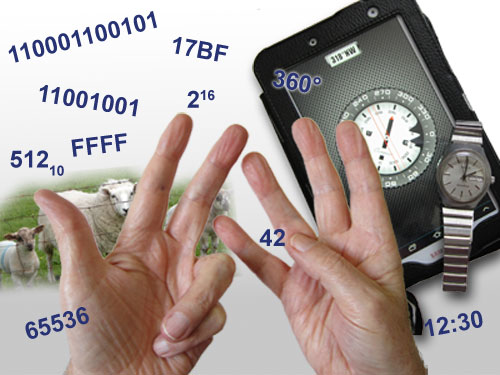# 1.0 Introduction to Number Systems## Why so many Number Systems?

Ask most people what the most commonly used number system is, and they would probably reply (after a bit of thought), the decimal system. But actually many number systems, and counting systems are used, without the users thinking much about it. For example clocks and compasses use the ancient Babylonian number system based on 60 rather than the decimal system based on 10. Why? Because 60 is easier to divide into equal segments, it can be evenly divided by 1,2,3,4,5,6,10,12,15, 20 and 30. This is much better for applications such as time, or degrees of angle than a base of 10, which can only be divided into equal parts by 1, 2 and 5.

Many counting systems are ancient in origin and are still in use because they are useful for particular purposes.

Using the decimal system it is easy to count up to ten fingers, using just the fingers on two hands. In northern Britain farmers, for centuries, used an ancient Celtic counting system, based on 20 (also called a score), to count their animals, and its use still persisted even into the second half of the twentieth century.

The binary system, based on 2, is just another special number system, and is used by digital electronic devices because digital circuits work on an electrical ‘on or off’ two state system, a number system based on 2 is therefore much easier for electronic devices to use. However binary is not a natural choice for human counting or calculation.

This module explains how binary, and some other number systems used in electronics work, and how computers and calculators use different forms of binary to carry out calculations. The important thing about this module is to get you to think about how the number systems work, so HOW the answer is achieved is more important than what the answer is.

Top of Page